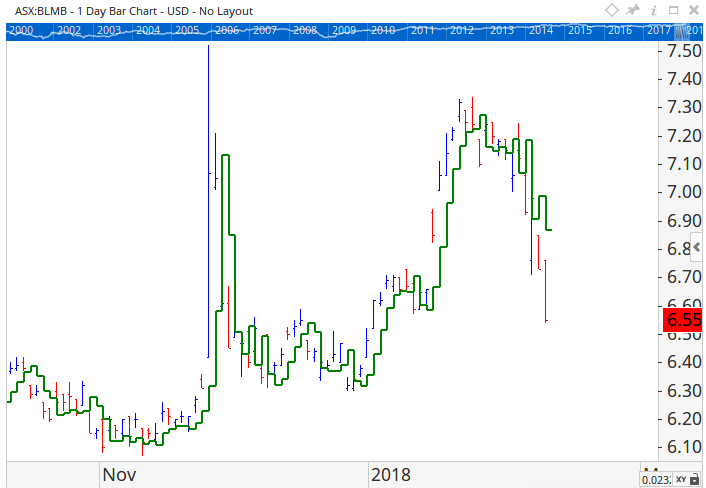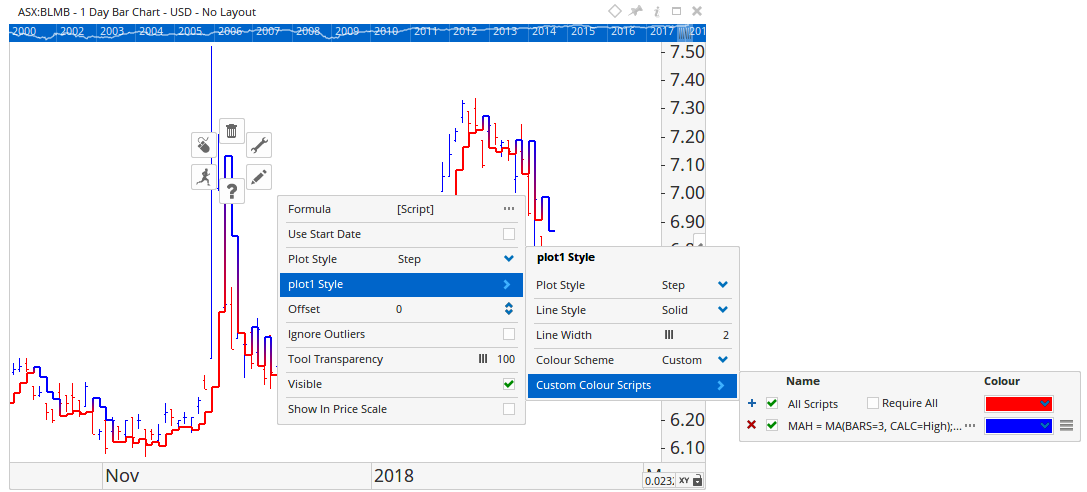Select Page

# Show Plot

Viewing 2 posts - 1 through 2 (of 2 total)
• Author
Posts
• #44703

Hi guys

I seek two 3 day simple MA’s plotted as stepped lines on the chart, one calculated with High, other Low; to be used as an entry and trailing stop indicator and plotted when close triggers an uptrend or down trend

show plot script
MAH = (Simple, high, 3 day);
MAL = (Simple, low, 3 day);

thought an if statement could decipher between plots but both lines are plotted

if(Close() < MAL, plot 1, plot 2);
plot 1 = MAH;
plot 2 = MAL

so I get both plots, and I’m guessing its just taking the plot definitions and not considering the if statement. I tried putting the plot def into the IF statement and get do lines…

#44707

Hi Randy,

The plot1 and plot2 lines will bypass the If statement and plot the functions they are assigned to.  If the idea is to swap between the Low / High MA you need just 1 plot, the IF function will handle the swap between the two.

MAH = MA(BARS=3, CALC=High);

MAL = MA(BARS=3, CALC=Low);

plot1=IF(Close() < MAL, MAH,MAL);

plot1.Plotstyle = Step;You can then setup the Show Plot to use a custom colour scheme so that when MAH is used it’s blue and MAL it’s red.The script used to set the blue colour was:

MAH = MA(BARS=3, CALC=High);

MAL = MA(BARS=3, CALC=Low);

C1 = IF(Close() < MAL, MAH,MAL);

MAH == C1

###### 1 user thanked author for this post.Thomas
Viewing 2 posts - 1 through 2 (of 2 total)
• You must be logged in to reply to this topic.